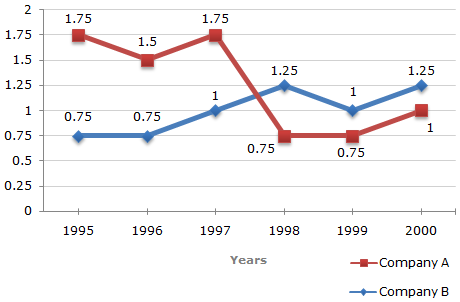# Data Interpretation - Line Charts

Exercise : Line Charts - Line Chart 7
Directions to Solve

Answer the questions based on the given line graph.

Ratio of Exports to Imports (in terms of money in Rs. crores) of Two Companies Over the Years1.
In how many of the given years were the exports more than the imports for Company A?
2
3
4
5
Explanation:

The exports are more than imports in those years for which the exports to imports ratio are more than 1. For Company A, such years are 1995, 1996 and 1997.

Thus, during these 3 years, the exports are more than the imports for Company A.

2.
If the imports of Company A in 1997 were increased by 40 percent, what would be the ratio of exports to the increased imports?
1.20
1.25
1.30
cannot be determined
Explanation:

In 1997 for Company A we have:

 E = 1.75     i.e.,     E = 1.75I I

where E amount of exports, I = amount of imports of Company A in 1997.

Now, the required imports I1 = I + 40% of I = 1.4I.Required ratio = E = 1.75I = 1.25. I1 1.4I

3.
If the exports of Company A in 1998 were Rs. 237 crores, what was the amount of imports in that year?
Rs. 189.6 crores
Rs. 243 crores
Rs. 281 crores
Rs. 316 crores
Explanation:

Let the amount of imports of Company A in 1998 be Rs. x crores.

 Then, 237 = 0.75x = 237 = 316. x 0.75Amount of imports of Company A in 1998 = Rs. 316 crores.

4.
In 1995, the export of Company A was double that of Company B. If the imports of Company A during the year was Rs. 180 crores, what was the approximate amount of imports pf Company B during that year?
Rs. 190 crores
Rs. 210 crores
Rs. 225 crores
Cannot be determined
Explanation:

In 1995 for Company A we have:

 EA = 1.75 ... (i) IA

[where EA = amount of exports, IA = amount of imports of Company a in 1995]

In 1995 for Company B we have:

 EB = 0.75 ... (ii) IB

[where EB = amount of exports, IB = amount of imports of Company B in 1995]

Also, we have EA = 2EB ... (iii)

Substituting IA = Rs. 180 crores (given) in (i), we get:

EA = Rs. (180 x 1.75) crores = Rs. 315 crores.

Using EA = Rs. 315 crores in (iii), we get:

 EB = EA = Rs.315crores. 2 2

 Substituting EB = Rs.315crores in (ii), we get: 2

 IB = EB = Rs.315crores = Rs. 210 crores. 0.75 2 x 0.75

i.e., amount of imports of Company B in 1995 = Rs. 210 crores.

5.
In which year(s) was the difference between impors and exports of Company B the maximum?
2000
1996
1998 and 2000
Cannot be determined
Explanation:

We shall try to find the difference between the imports and exports of Company B for various years one by one:

For 1995: We have

 E = 0.75 I

where E = amount of exports, I = amount of imports in 1995.E = 0.75II - E = 0.75 x I = 0.25I.

Thus, the difference between the imports and exports of Company B in 1995 is dependent on the amount of imports of Company B in 1995.

Similarly, the difference for other years can be determined only if the amount of imports for these years is known.

Since the imports or exports for various years are not know, the differences between and exports for various years cannot be determined.